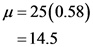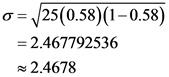# In a 1993 Gallo poll, it was noted that 58% of adult Americansconsider human cloning to be morally wrong

In a 1993 Gallo poll, it was noted that 58% of adult Americansconsider human cloning to be morally wrong. Assuming that theresult of this poll is till true for the current population ofadult Americans, find the mean and standard deviation of theprobability distribution of those who share the view based a sampleof 25 adult Americans. Give the results of the experiment perrepetition.

From the given information we have n=25 and p=0.58
The mean of the probability distribution of those who share the view based on a sample of 25 adult Americans is,The mean of the binomial distribution is 14.5.
The value of the mean is what we expect to obtain, one average, per repetition of the binomial experiment. For, instance, if we select, many samples of 25 adults each, we expect that each sample will contain an average of 14.5 adults. On average 14.5 adult Americans consider human cloning to be morally wrong.

The standard deviation of the probability distribution of those who share the view based on a sample of 25 adult Americans is,The standard deviation value is 2.4678.
The standard deviation for the given data is about 2.4678. Values that are more than two standard deviations from the mean are considered as unusual.

The mean of the binomial distribution is 14.5.
The standard deviation value is 2.4678.